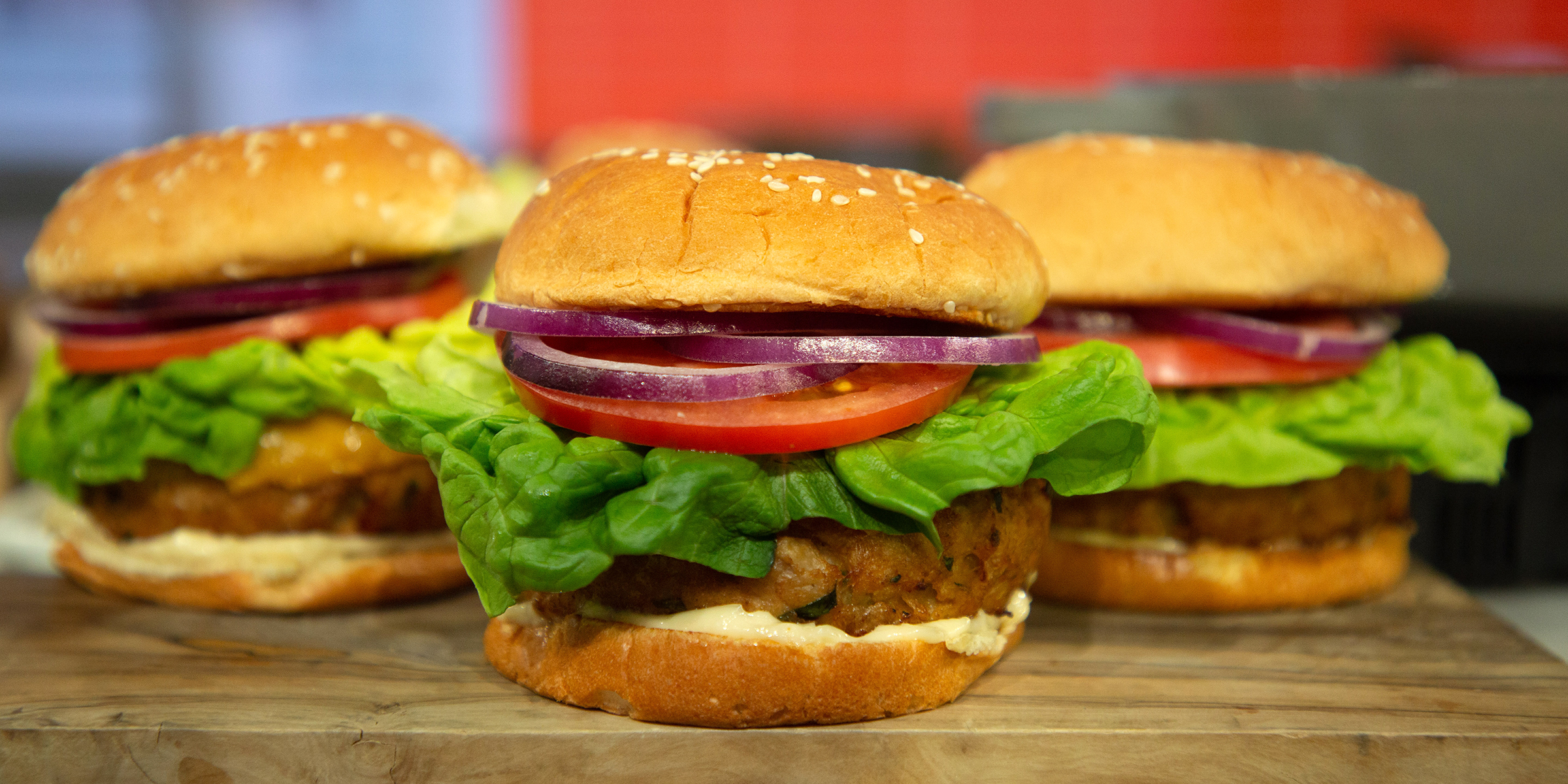from today.com

Overview

In this practical you’ll practice plotting data with the amazing ggplot2 package. By the end of this practical you will know how to:

1. Build a plot step-by-step.
2. Use multiple geoms.
3. Work with facets.
5. Create image files.

A - Setup

1. Open your BaselRBootcamp R project. It should already have the folders 1_Data and 2_Code. Make sure that the data files listed in the Datasets section above are in your 1_Data folder.
# Done!
1. Open a new R script. At the top of the script, using comments, write your name and the date and “Plotting Practical”.
## NAME
## DATE
## Plotting Practical
1. Save the file under the name plotting_practical.R in the 2_Code folder.

2. Using library() load the tidyverse and ggthemes packages for this practical listed in the Functions section above. If you don’t have them installed, you’ll need to install them, see the Functions tab above for installation instructions.

library(tidyverse)
library(ggthemes)
library(tidyverse)
library(ggthemes)
1. For this practical, we’ll use the mcdonalds.csv data set, which contains nutrition information about items from McDonalds. Using read_csv(), load the data into R and store it as a new object called mcdonalds.
# Load mcdonalds.csv as a new object called mcdonalds
1. Using print(), summary(), head(), and View(), explore the data to make sure it was loaded correctly.
mcdonalds
# A tibble: 260 x 14
Category Item  ServingSize Calories CaloriesfromFat TotalFat
<chr>    <chr> <chr>          <dbl>           <dbl>    <dbl>
1 Breakfa… Egg … 4.8 oz (13…      300             120       13
2 Breakfa… Egg … 4.8 oz (13…      250              70        8
3 Breakfa… Saus… 3.9 oz (11…      370             200       23
4 Breakfa… Saus… 5.7 oz (16…      450             250       28
5 Breakfa… Saus… 5.7 oz (16…      400             210       23
6 Breakfa… Stea… 6.5 oz (18…      430             210       23
7 Breakfa… Baco… 5.3 oz (15…      460             230       26
8 Breakfa… Baco… 5.8 oz (16…      520             270       30
9 Breakfa… Baco… 5.4 oz (15…      410             180       20
10 Breakfa… Baco… 5.9 oz (16…      470             220       25
# … with 250 more rows, and 8 more variables: SaturatedFat <dbl>,
#   TransFat <dbl>, Cholesterol <dbl>, Sodium <dbl>, Carbohydrates <dbl>,
#   DietaryFiber <dbl>, Sugars <dbl>, Protein <dbl>
summary(mcdonalds)
Category             Item           ServingSize           Calories
Length:260         Length:260         Length:260         Min.   :   0
Class :character   Class :character   Class :character   1st Qu.: 210
Mode  :character   Mode  :character   Mode  :character   Median : 340
Mean   : 368
3rd Qu.: 500
Max.   :1880
CaloriesfromFat    TotalFat      SaturatedFat      TransFat
Min.   :   0    Min.   :  0.0   Min.   : 0.00   Min.   :0.000
1st Qu.:  20    1st Qu.:  2.4   1st Qu.: 1.00   1st Qu.:0.000
Median : 100    Median : 11.0   Median : 5.00   Median :0.000
Mean   : 127    Mean   : 14.2   Mean   : 6.01   Mean   :0.204
3rd Qu.: 200    3rd Qu.: 22.2   3rd Qu.:10.00   3rd Qu.:0.000
Max.   :1060    Max.   :118.0   Max.   :20.00   Max.   :2.500
Cholesterol      Sodium     Carbohydrates    DietaryFiber
Min.   :  0   Min.   :   0   Min.   :  0.0   Min.   :0.00
1st Qu.:  5   1st Qu.: 108   1st Qu.: 30.0   1st Qu.:0.00
Median : 35   Median : 190   Median : 44.0   Median :1.00
Mean   : 55   Mean   : 496   Mean   : 47.3   Mean   :1.63
3rd Qu.: 65   3rd Qu.: 865   3rd Qu.: 60.0   3rd Qu.:3.00
Max.   :575   Max.   :3600   Max.   :141.0   Max.   :7.00
Sugars         Protein
Min.   :  0.0   Min.   : 0.0
1st Qu.:  5.8   1st Qu.: 4.0
Median : 17.5   Median :12.0
Mean   : 29.4   Mean   :13.3
3rd Qu.: 48.0   3rd Qu.:19.0
Max.   :128.0   Max.   :87.0
# A tibble: 6 x 14
Category Item  ServingSize Calories CaloriesfromFat TotalFat SaturatedFat
<chr>    <chr> <chr>          <dbl>           <dbl>    <dbl>        <dbl>
1 Breakfa… Egg … 4.8 oz (13…      300             120       13            5
2 Breakfa… Egg … 4.8 oz (13…      250              70        8            3
3 Breakfa… Saus… 3.9 oz (11…      370             200       23            8
4 Breakfa… Saus… 5.7 oz (16…      450             250       28           10
5 Breakfa… Saus… 5.7 oz (16…      400             210       23            8
6 Breakfa… Stea… 6.5 oz (18…      430             210       23            9
# … with 7 more variables: TransFat <dbl>, Cholesterol <dbl>,
#   Sodium <dbl>, Carbohydrates <dbl>, DietaryFiber <dbl>, Sugars <dbl>,
#   Protein <dbl>
# View(kc_house)

B - Building a plot step-by-step

In this section, you’ll build the following plot step by step.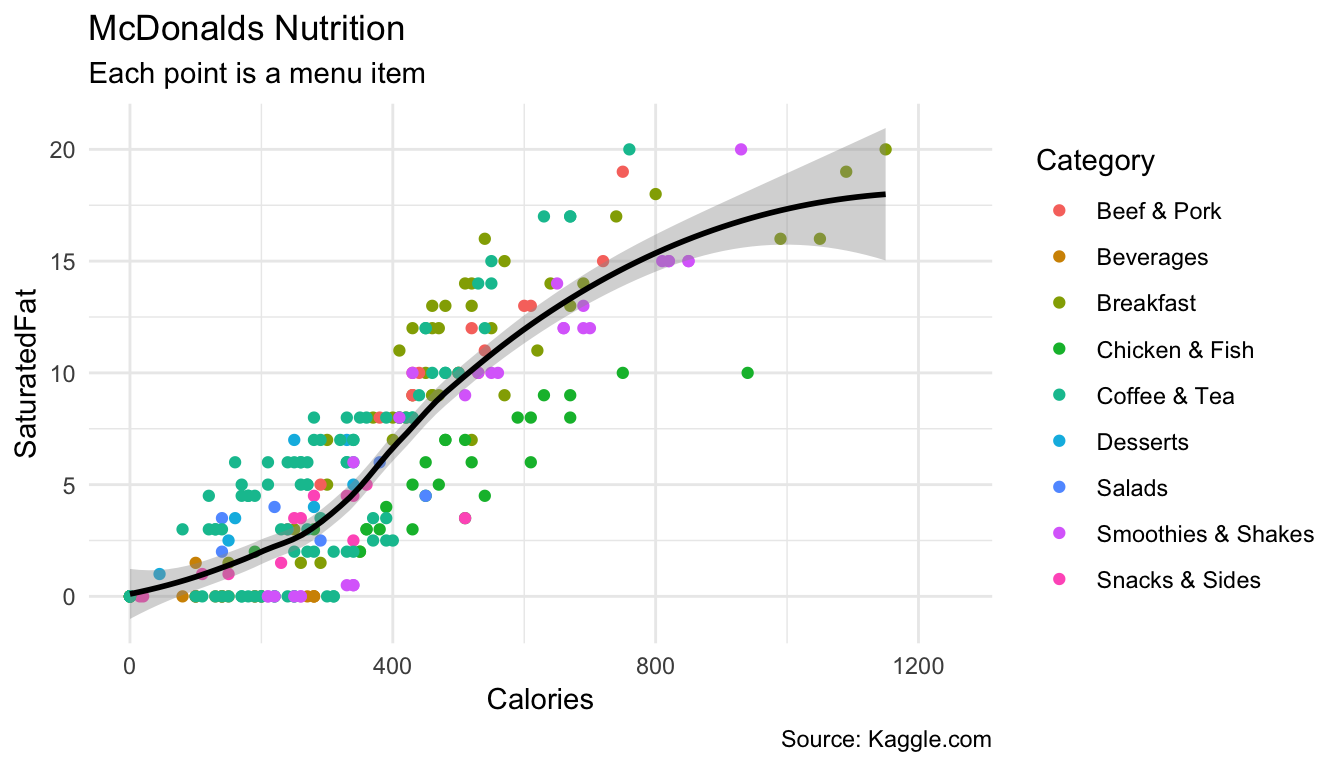1. Using ggplot(), create the following blank plot using the data and mapping arguments (but no geom). Use Calories for the x aesthetic and SaturatedFat for the y aesthetic
ggplot(data = mcdonalds,
mapping = aes(x = XX, y = XX))
ggplot(mcdonalds, aes(x = Calories, y = SaturatedFat))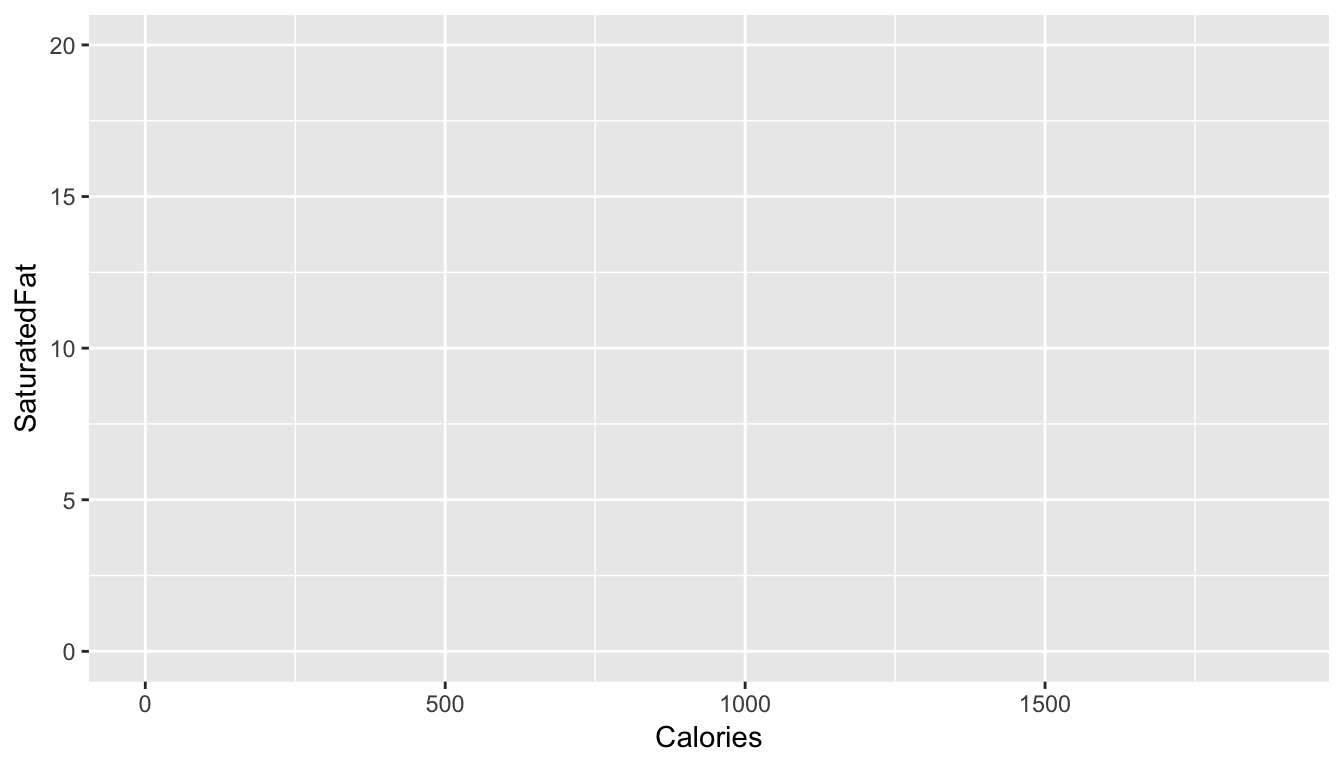1. Using geom_point(), add points to the plot
ggplot(data = mcdonalds,
mapping = aes(x = XX, y = XX)) +
geom_point()
ggplot(mcdonalds, aes(x = Calories, y = SaturatedFat)) +
geom_point()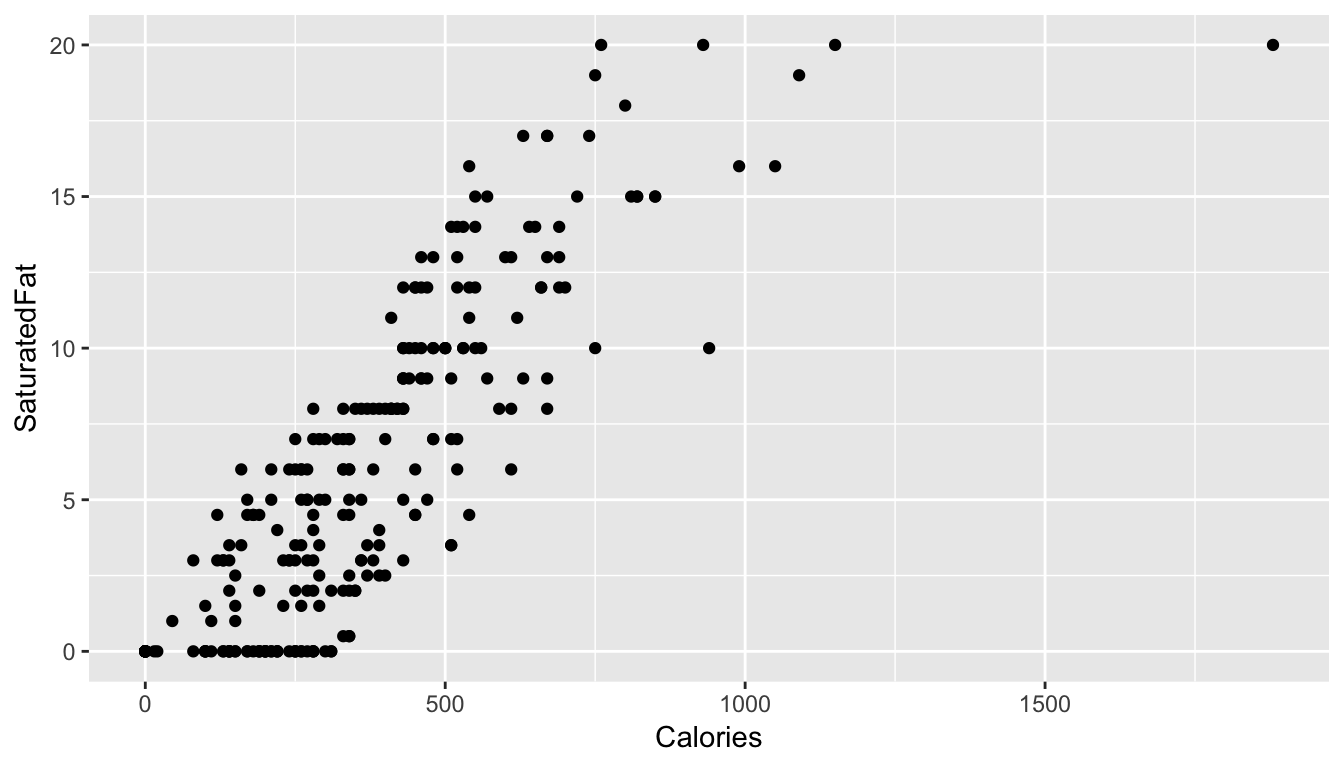1. Using the color aesthetic mapping, color the points by their Category.
ggplot(mcdonalds, aes(x = XX, y = XX, col = XX)) +
geom_point()
ggplot(mcdonalds, aes(x = Calories, y = SaturatedFat, col = Category)) +
geom_point()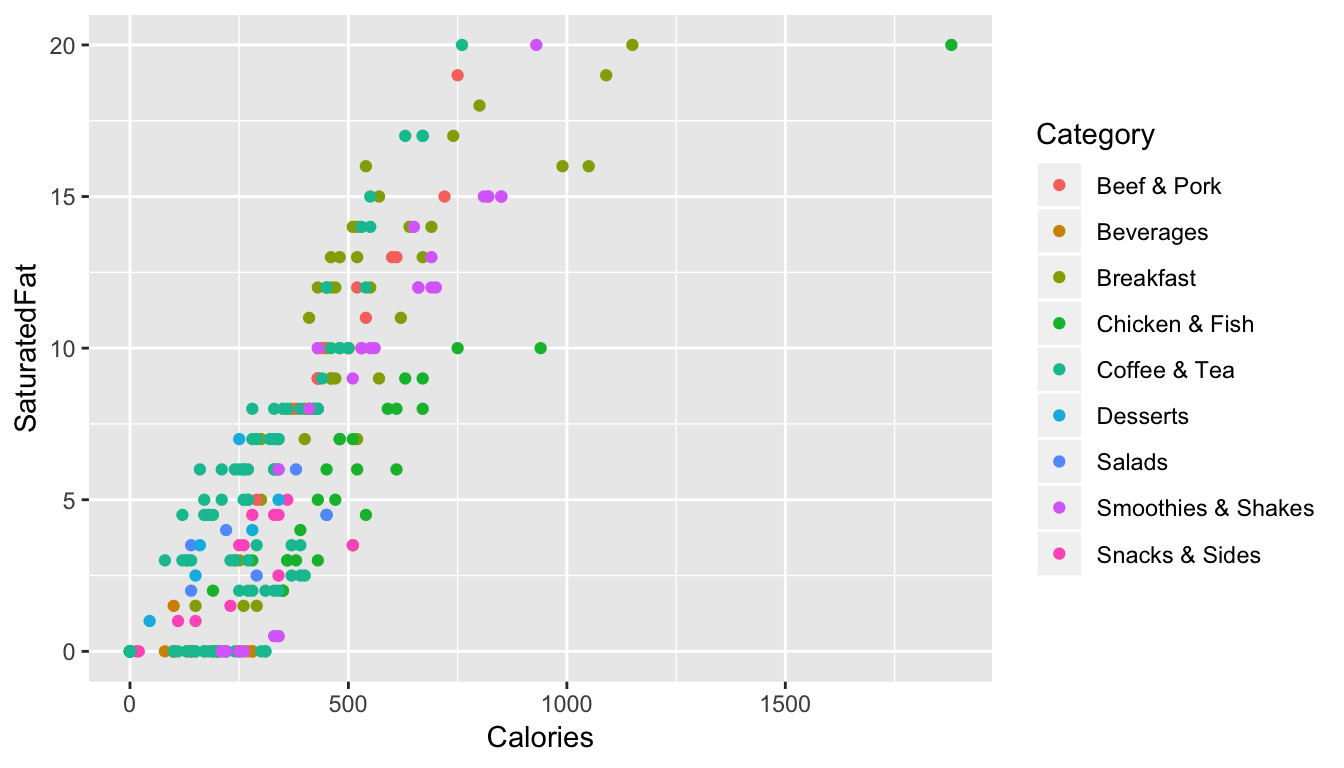1. Add a smoothed average line using geom_smooth().
ggplot(mcdonalds, aes(x = XX, y = XX, col = XX)) +
geom_point() +
geom_smooth()
ggplot(mcdonalds, aes(x = Calories, y = SaturatedFat, col = Category)) +
geom_point() +
geom_smooth()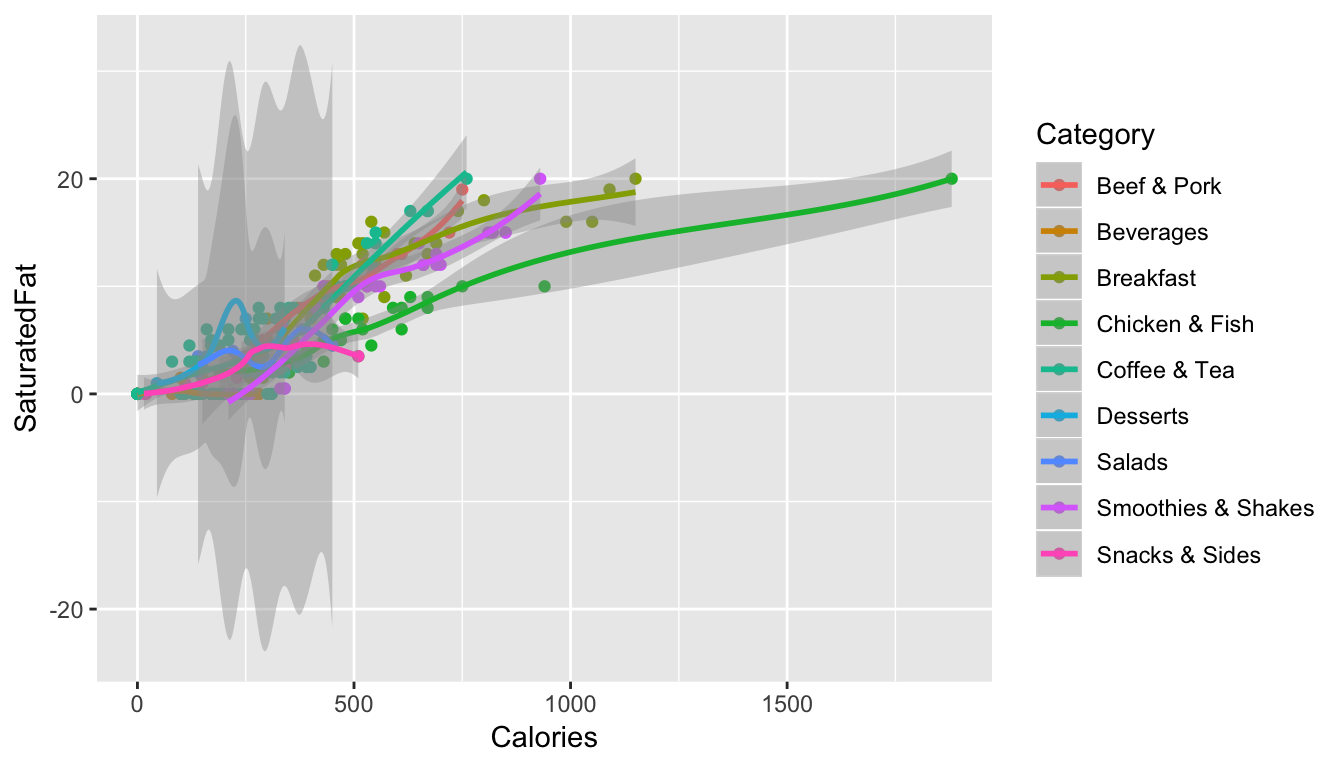1. Oops! Did you get several smoothed lines instead of just one? Fix this by specifying that the line should have one color: "black". When you do, you should then only see one line.
ggplot(mcdonalds, aes(x = XX, y = XX, col = XX)) +
geom_point() +
geom_smooth(col = "XX")
ggplot(mcdonalds, aes(x = Calories, y = SaturatedFat, col = Category)) +
geom_point() +
geom_smooth(col = "black")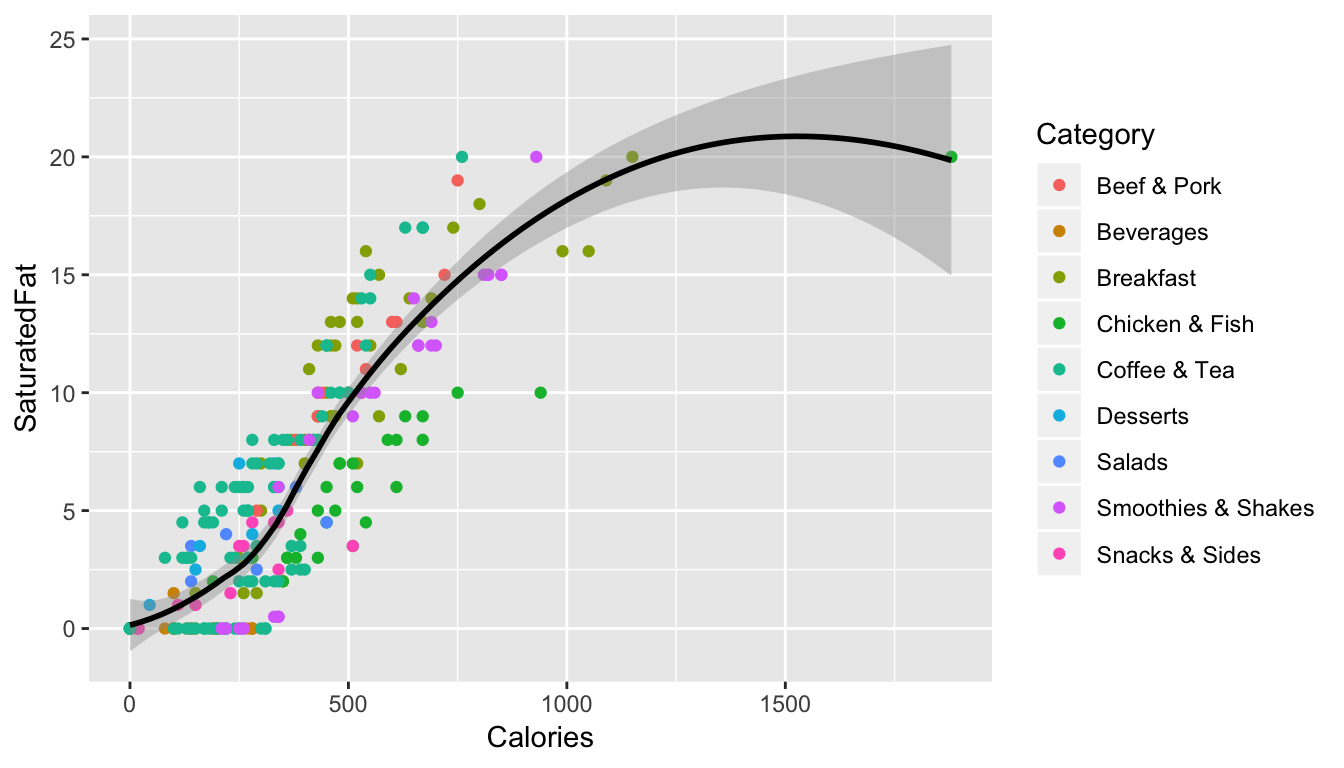1. Add appropriate labels using the labs() function.
ggplot(mcdonalds, aes(x = XX, y = XX, col = XX)) +
geom_point() +
geom_smooth(col = "XX") +
labs(title = "XX",
subtitle = "XX",
caption = "XX")
ggplot(mcdonalds, aes(x = Calories, y = SaturatedFat, col = Category)) +
geom_point() +
geom_smooth(col = "black") +
labs(title = "McDonalds Nutrition",
subtitle = "Each point is a menu item",
caption = "Source: Kaggle.com")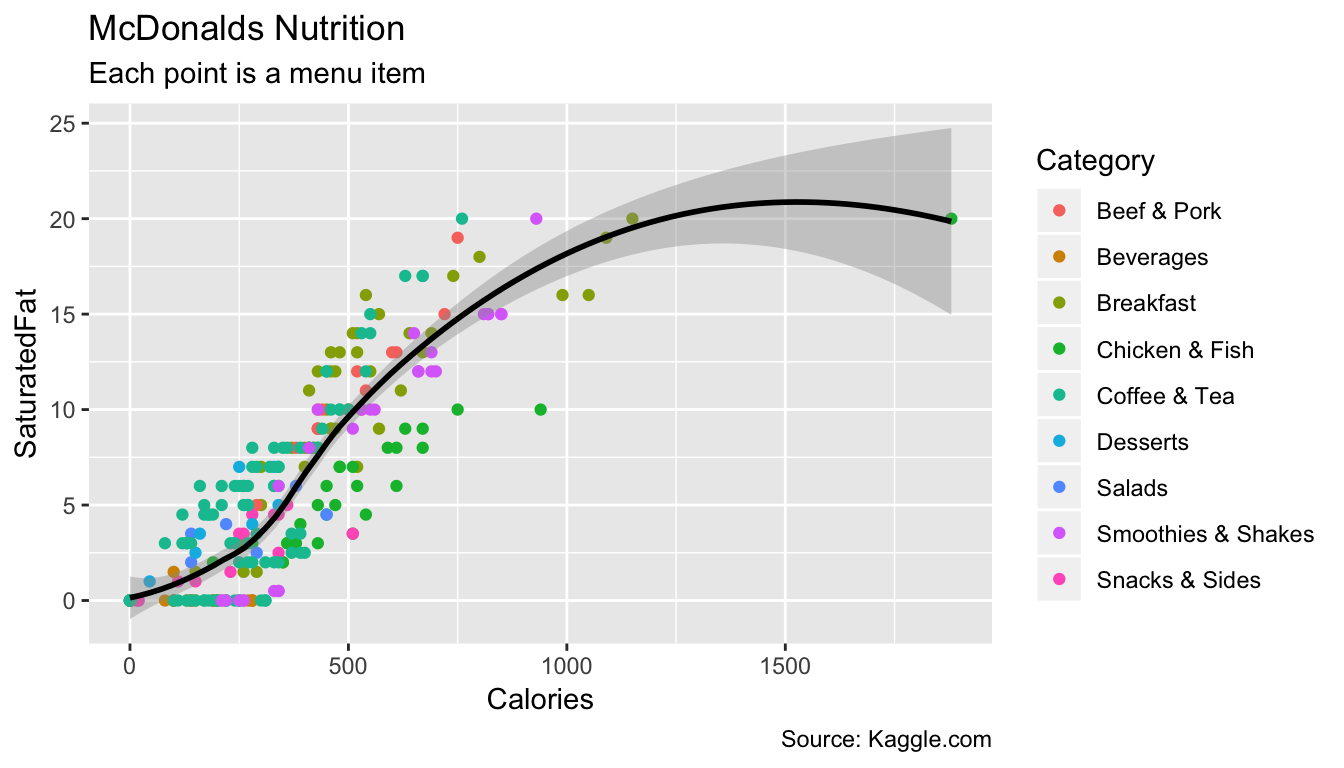1. Set the limits of the x-axis to 0 and 1250 using xlim().
ggplot(mcdonalds, aes(x = XX, y = XX, col = XX)) +
geom_point() +
geom_smooth(col = "XX") +
labs(title = "XX",
subtitle = "XX",
caption = "XX") +
xlim(XX, XX)
ggplot(mcdonalds, aes(x = Calories, y = SaturatedFat, col = Category)) +
geom_point() +
geom_smooth(col = "black") +
labs(title = "McDonalds Nutrition",
subtitle = "Each point is a menu item",
caption = "Source: Kaggle.com") +
xlim(0, 1250)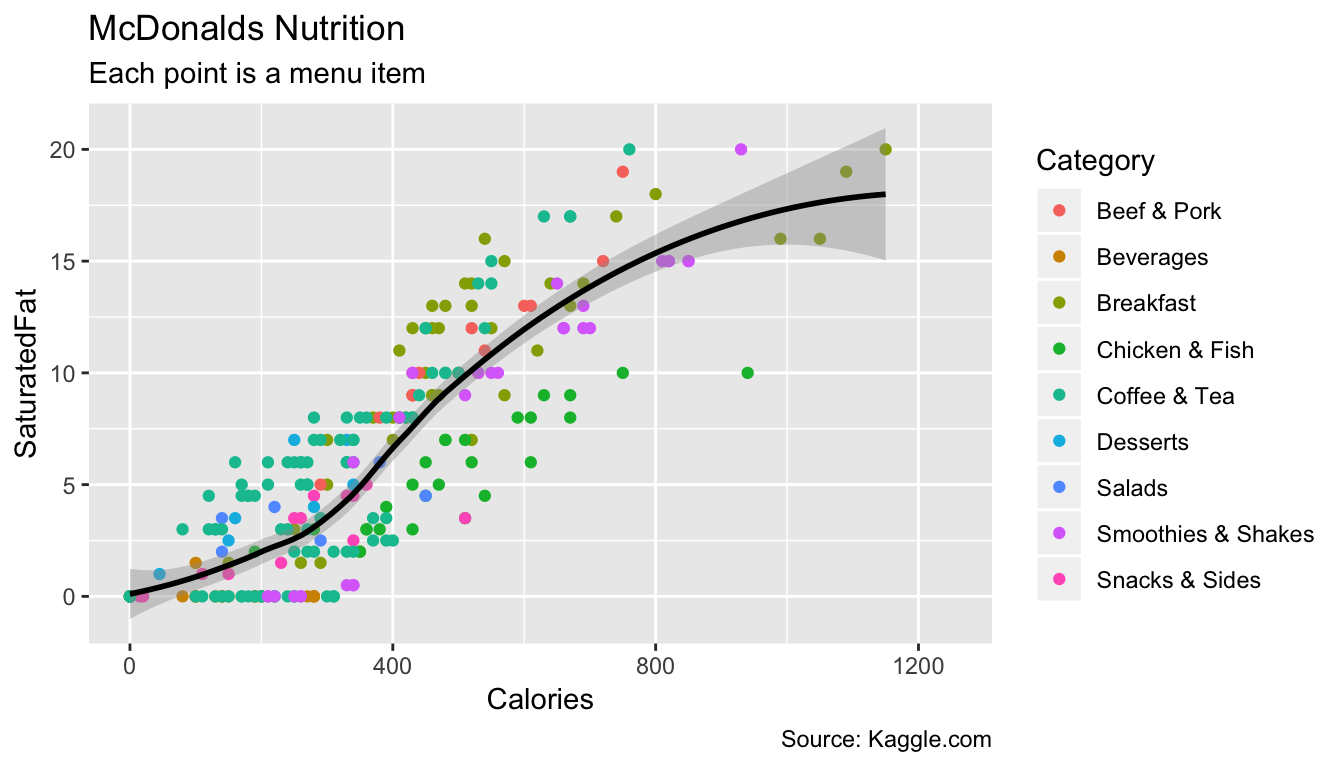1. Finally, set the plotting theme to theme_minimal(). You should now have the final plot!
ggplot(mcdonalds, aes(x = XX, y = XX, col = XX)) +
geom_point() +
geom_smooth(col = "XX") +
labs(title = "XX",
subtitle = "XX",
caption = "XX")+
xlim(XX, XX) +
theme_minimal()
ggplot(mcdonalds, aes(x = Calories, y = SaturatedFat, col = Category)) +
geom_point() +
geom_smooth(col = "black") +
labs(title = "McDonalds Nutrition",
subtitle = "Each point is a menu item",
caption = "Source: Kaggle.com") +
xlim(0, 1250) +
theme_minimal()C - Adding multiple geoms

1. Create the following plot showing the relationship between menu category and calories
ggplot(data = mcdonalds, aes(x = XX, y = XX, fill = XX)) +
geom_violin() +
guides(fill = FALSE) +
labs(title = "XX",
subtitle = "XX")
ggplot(data = mcdonalds, aes(x = Category, y = Calories, fill = Category)) +
geom_violin() +
guides(fill = FALSE) +
labs(title = "McDonalds",
subtitle = "Calorie distribution by menu category")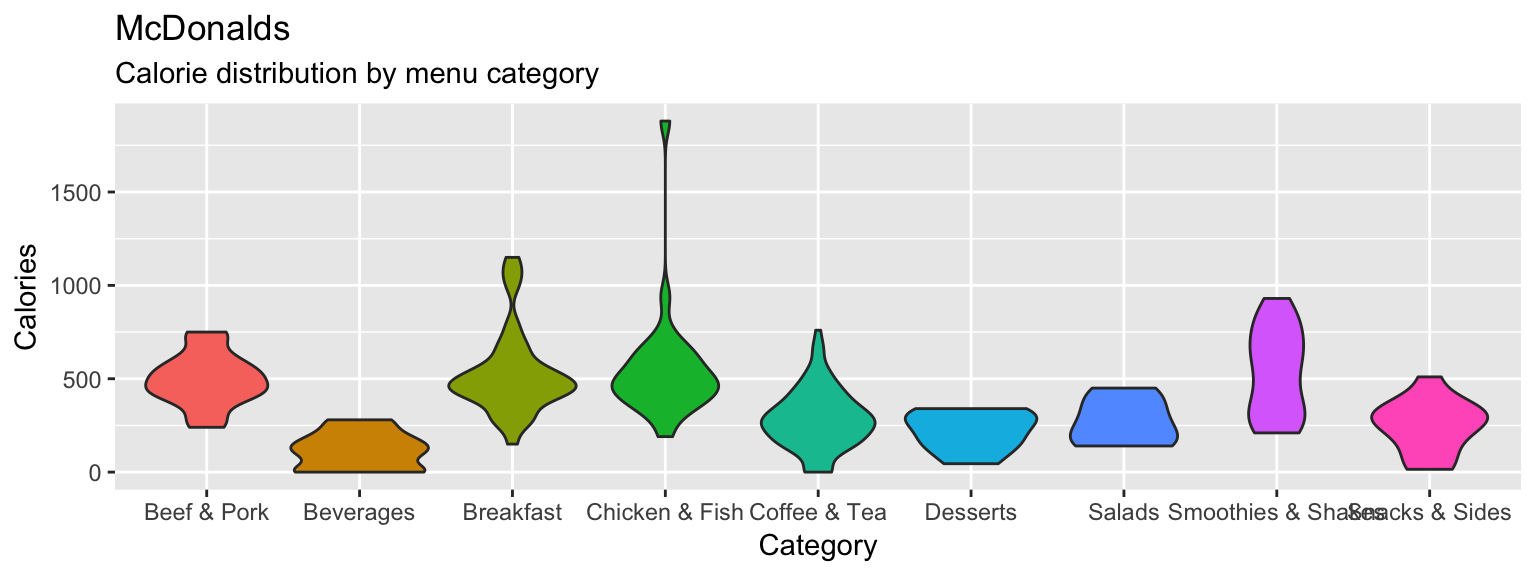1. Include the additional argument + stat_summary(fun.y = "mean", geom = "point", col = "white", size = 4) to include points showing the mean of each distribution
ggplot(data = mcdonalds, aes(x = Category, y = Calories, fill = Category)) +
geom_violin() +
guides(fill = FALSE) +
stat_summary(fun.y = "mean", geom = "point", col = "white", size = 4) +
labs(title = "McDonalds",
subtitle = "Calorie distribution by menu category")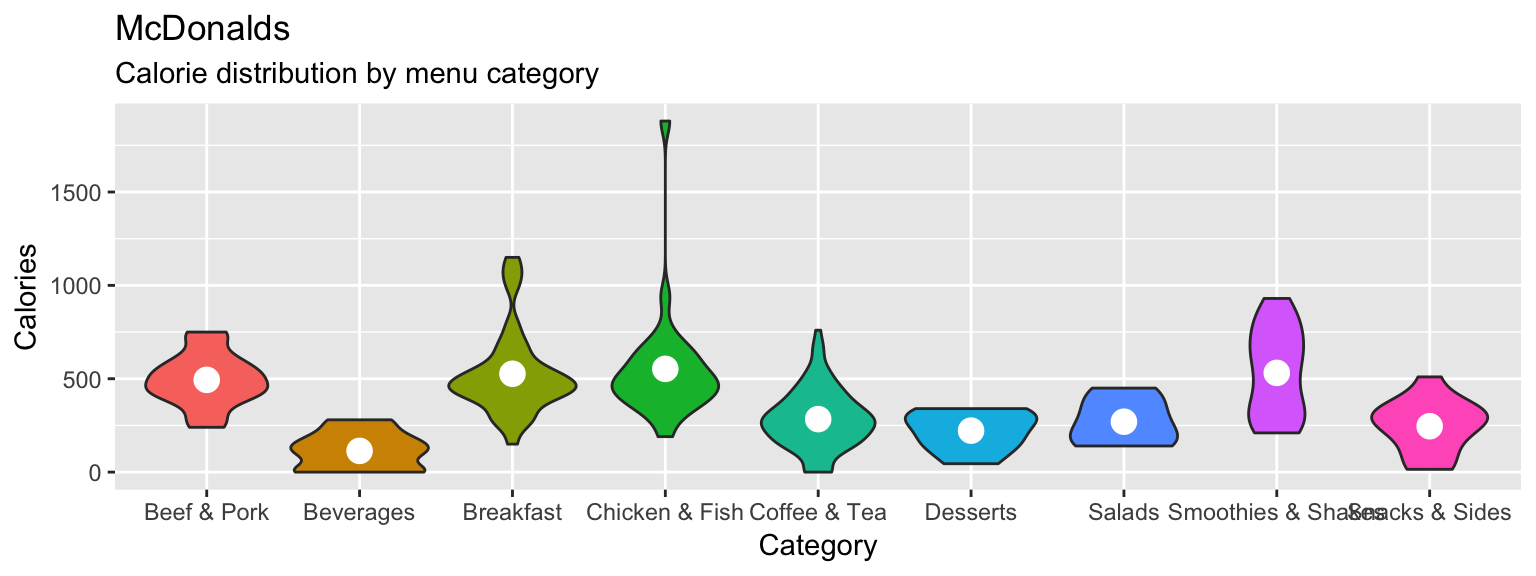1. Now add + geom_jitter(width = .1, alpha = .5) to your plot, what do you see?
ggplot(data = mcdonalds, aes(x = Category, y = Calories, fill = Category)) +
geom_violin() +
geom_jitter(width = .1, alpha = .5) +
guides(fill = FALSE) +
stat_summary(fun.y = "mean", geom = "point", col = "white", size = 4) +
labs(title = "McDonalds",
subtitle = "Calorie distribution by menu category")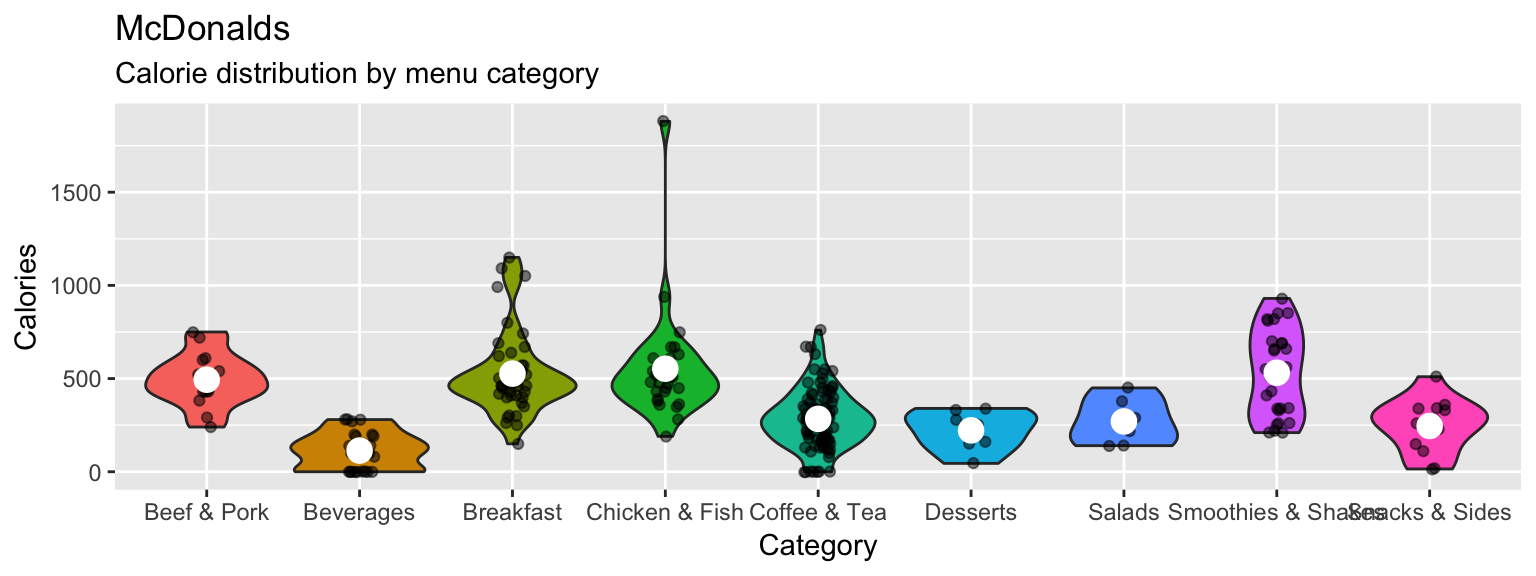1. Play around with your plotting arguments to see how the results change! Each time you make a change, run the plot again to see your new output!
• Change the summary function in stat_summary() from "mean" to "median".
• Change the size of the points in stat_summary() to something much bigger (or smaller).
• Change the width argument in geom_jitter() to width = 0.
• Instead of using geom_violin(), try geom_boxplot().
• Remove the fill = Category aesthetic entirely.
ggplot(data = mcdonalds, aes(x = Category, y = Calories)) +
geom_boxplot() +
geom_jitter(width = 0, alpha = .5) +
guides(fill = FALSE) +
stat_summary(fun.y = "median", geom = "point", col = "white", size = 10) +
labs(title = "McDonalds",
subtitle = "Calorie distribution by menu category")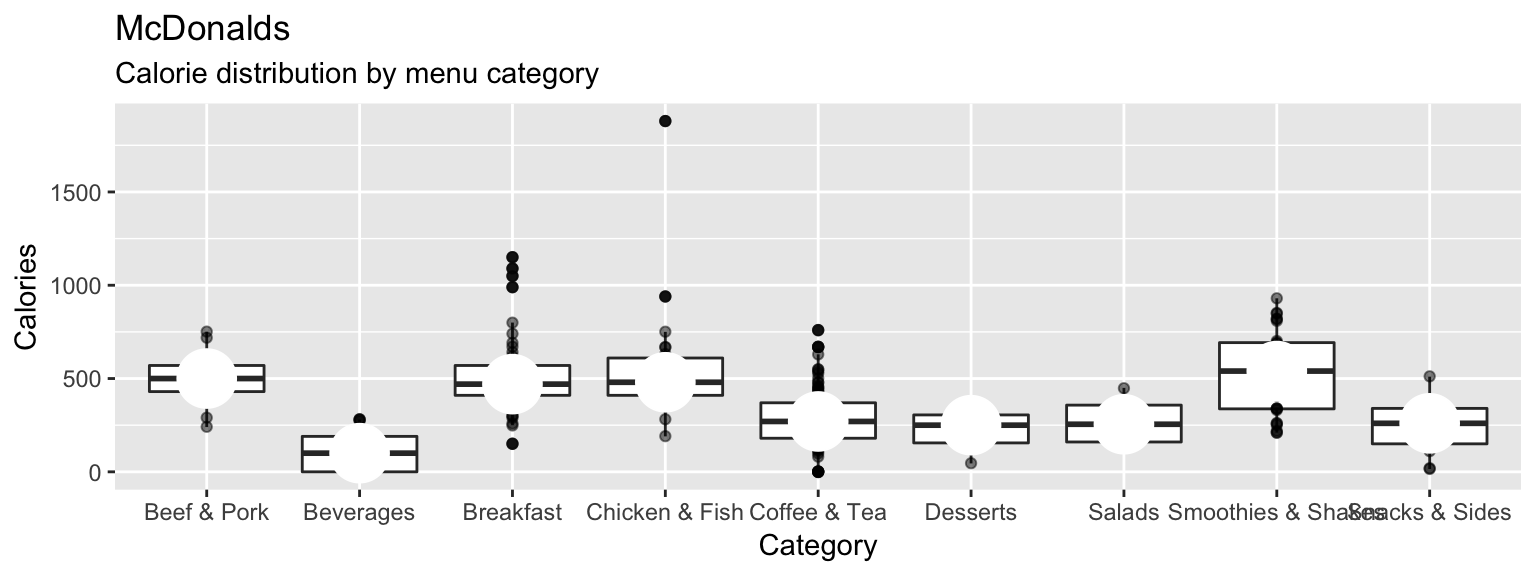D - Using facets

1. Create the following plot showing the relationship between Sodium and Calories.
ggplot(XX, aes(x = XX, y = XX)) +
geom_point(alpha = .2) +
facet_wrap(~ XX) +
labs(title = "XX",
subtitle = "XX") +
theme_minimal()
ggplot(mcdonalds, aes(x = Sodium, y = Calories)) +
geom_point(alpha = .2) +
facet_wrap(~Category) +
labs(title = "McDonales",
subtitle = "Sodium vs. Calories") +
theme_minimal()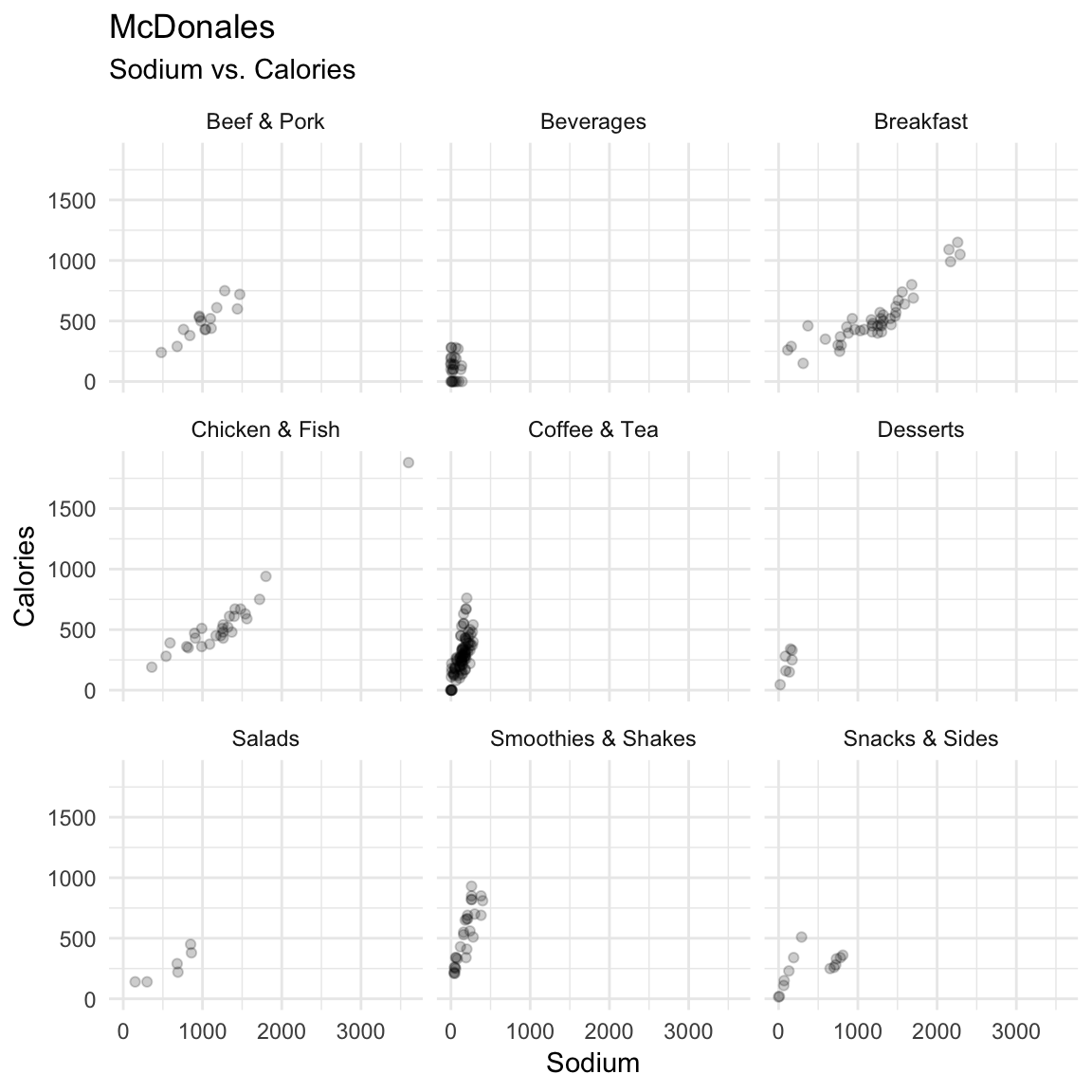1. Try the following ways to customise your plot:
• Color the points by Category.
• Add a smoothed line to each plot with geom_smooth().
ggplot(mcdonalds, aes(x = Sodium, y = Calories, fill = Category)) +
geom_point(alpha = .2) +
facet_wrap(~Category) +
labs(title = "McDonales",
subtitle = "Sodium vs. Calories") +
geom_smooth() +
theme_minimal()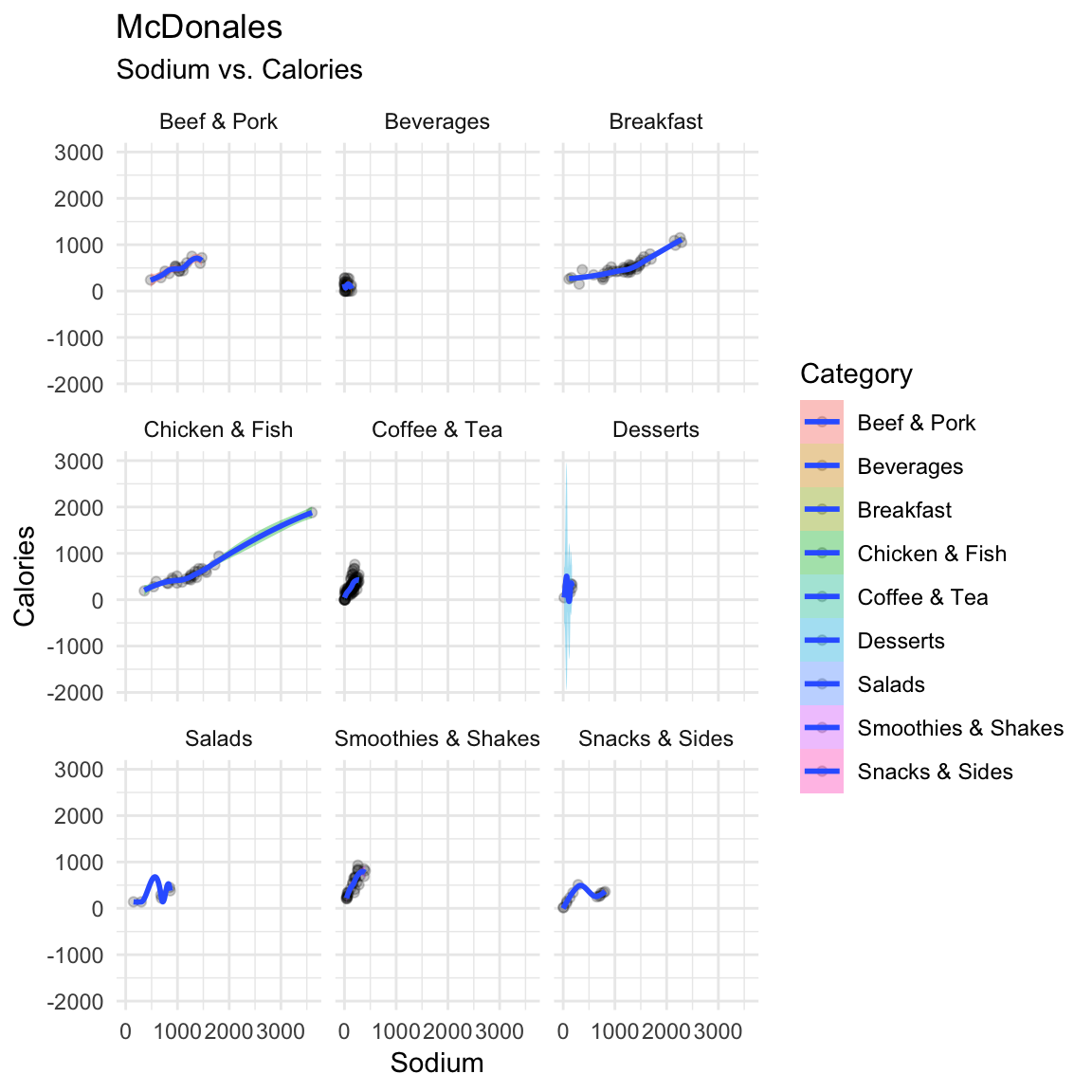E - Adjusting colors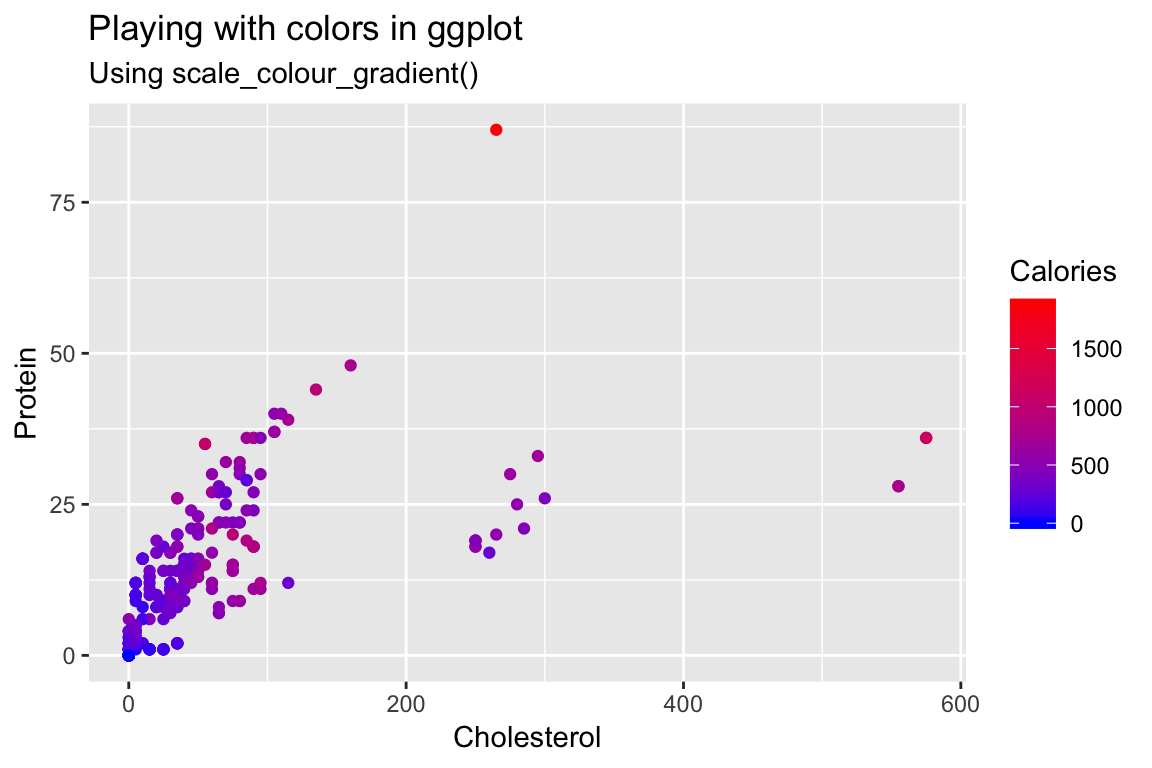1. Create a scatterplot showing the relationship between Cholesterol and Protein .

2. Color the points according to their Calories by specifying the col aesthetic.

3. Change the colors by including the additional argument + scale_colour_gradient(low = "blue", high = "red").

4. Customize! Look at all of the named colors in R by running colors(). Then, use two new colors in your plot.

ggplot(mcdonalds, aes(x = Cholesterol,
y = Protein,
col = Calories)) +
geom_point() +
scale_colour_gradient(low = "tomato2", high = "steelblue1") +
labs(title = "Playing with colors in ggplot",
subtitle = "Using scale_colour_gradient()")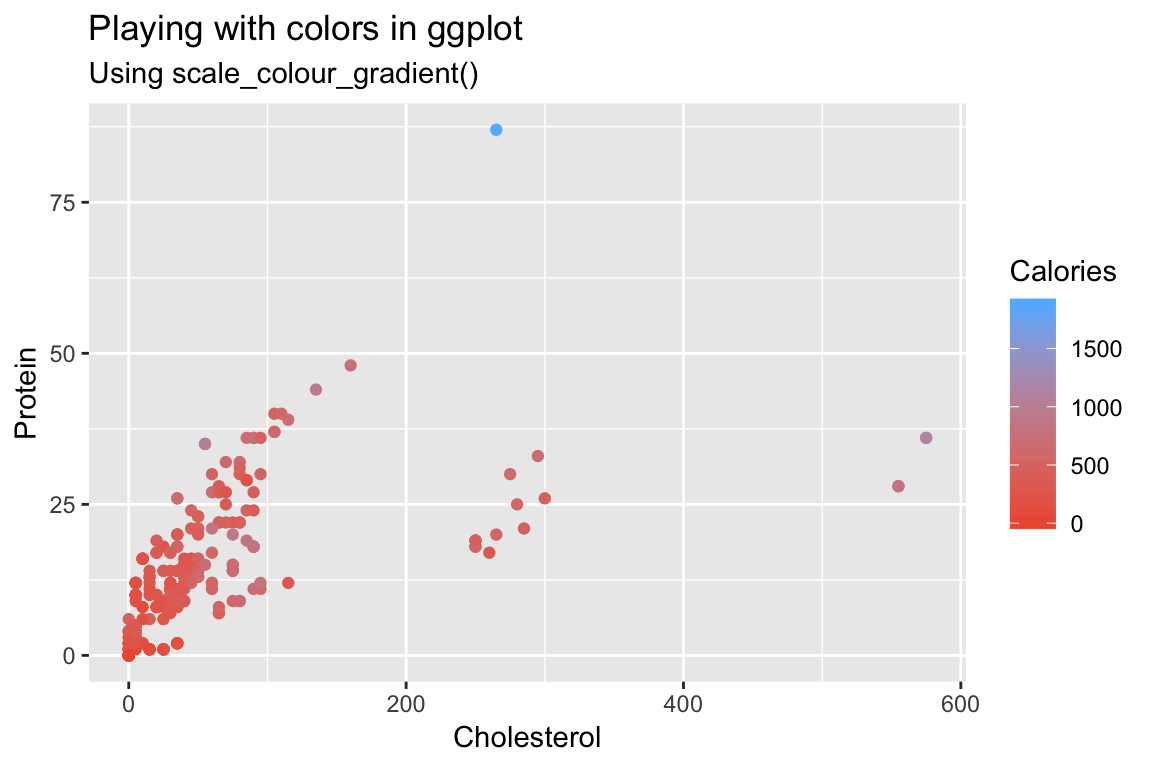F - Summary statistics

1. Create the following plot showing the mean number of calories for each menu category using the following template:
ggplot(XX, aes(x = XX, y = X)) +
stat_summary(geom = "bar",
fun.y = "mean") +
labs(title = "XX",
subtitle = "XX")
ggplot(mcdonalds, aes(x = Category, y = Calories)) +
stat_summary(geom = "bar",
fun.y = "mean") +
labs(title = "Calories by McDonalds menu category",
subtitle = "Bars represent means")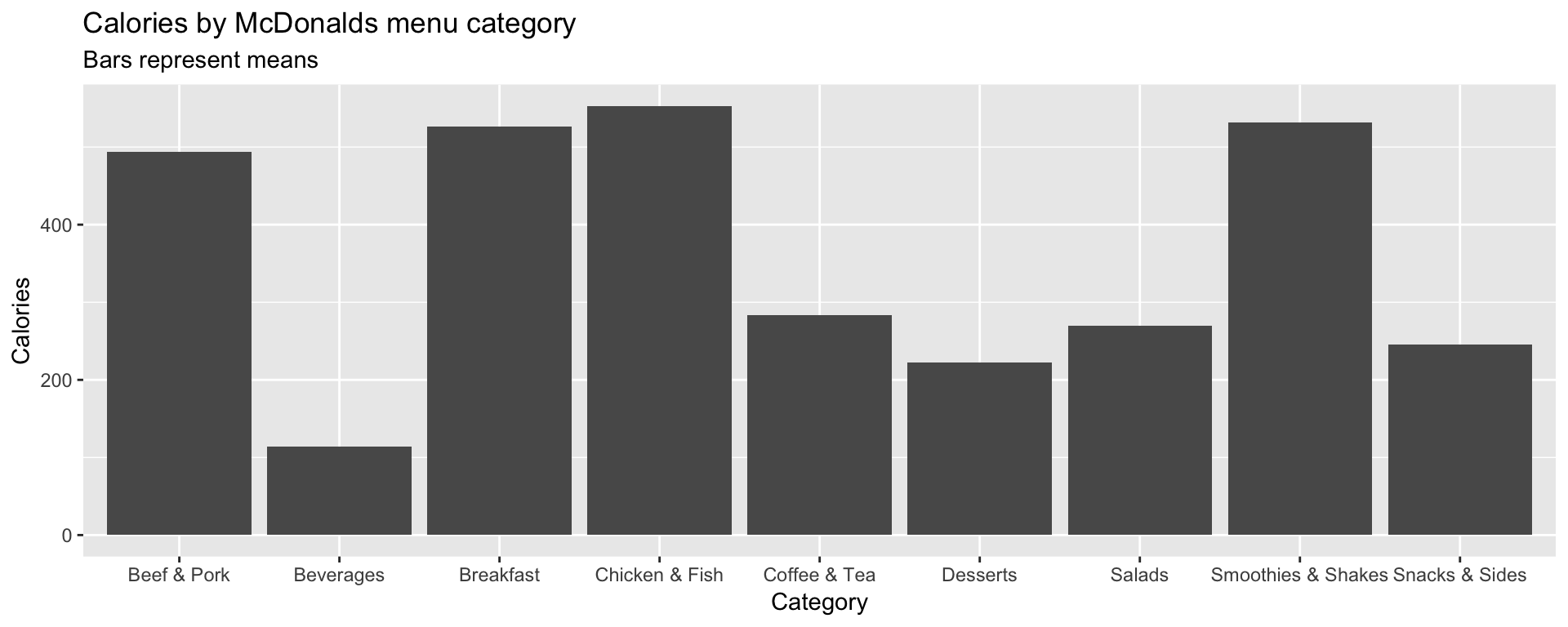ggplot(mcdonalds, aes(x = Category, y = Calories)) +
stat_summary(geom = "bar",
fun.y = "mean") +
labs(title = "Calories by McDonalds menu category",
subtitle = "Bars represent means")1. Customize your plot!
• Instead of showing the "mean", show the "median".
• Give each bar a different color.
• Add overlapping points showing the individual items using geom_point(), geom_count() or geom_jitter().
ggplot(mcdonalds, aes(x = Category, y = Calories, col = Category)) +
stat_summary(geom = "bar",
fun.y = "median") +
geom_jitter() +
labs(title = "Calories by McDonalds menu category",
subtitle = "Bars represent means")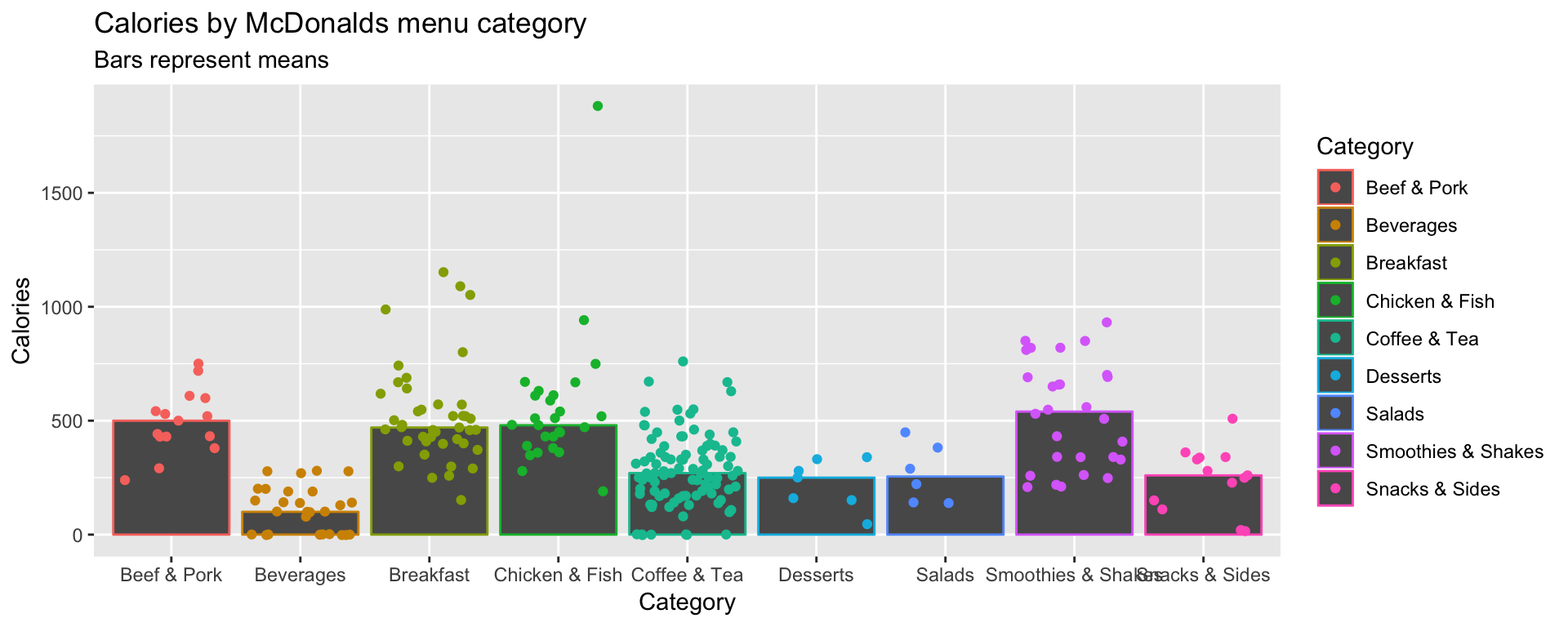G - Saving plots

1. It’s time to save your favorite plot to an image file! Pick your favorite plot you’ve created so far. Then, assign the plot to a new object called mcdonalds_gg using mcdonalds_gg <- ggplot(...)
mcdonalds_gg <- ggplot(...) + ... # Include your plotting code here
mcdonalds_gg <- ggplot(mcdonalds, aes(x = Category, y = Calories, col = Category)) +
stat_summary(geom = "bar",
fun.y = "median") +
geom_jitter() +
labs(title = "Calories by McDonalds menu category",
subtitle = "Bars represent means")
1. Evaluate your mcdonalds_gg object to see that it does indeed contain your plot.
mcdonalds_gg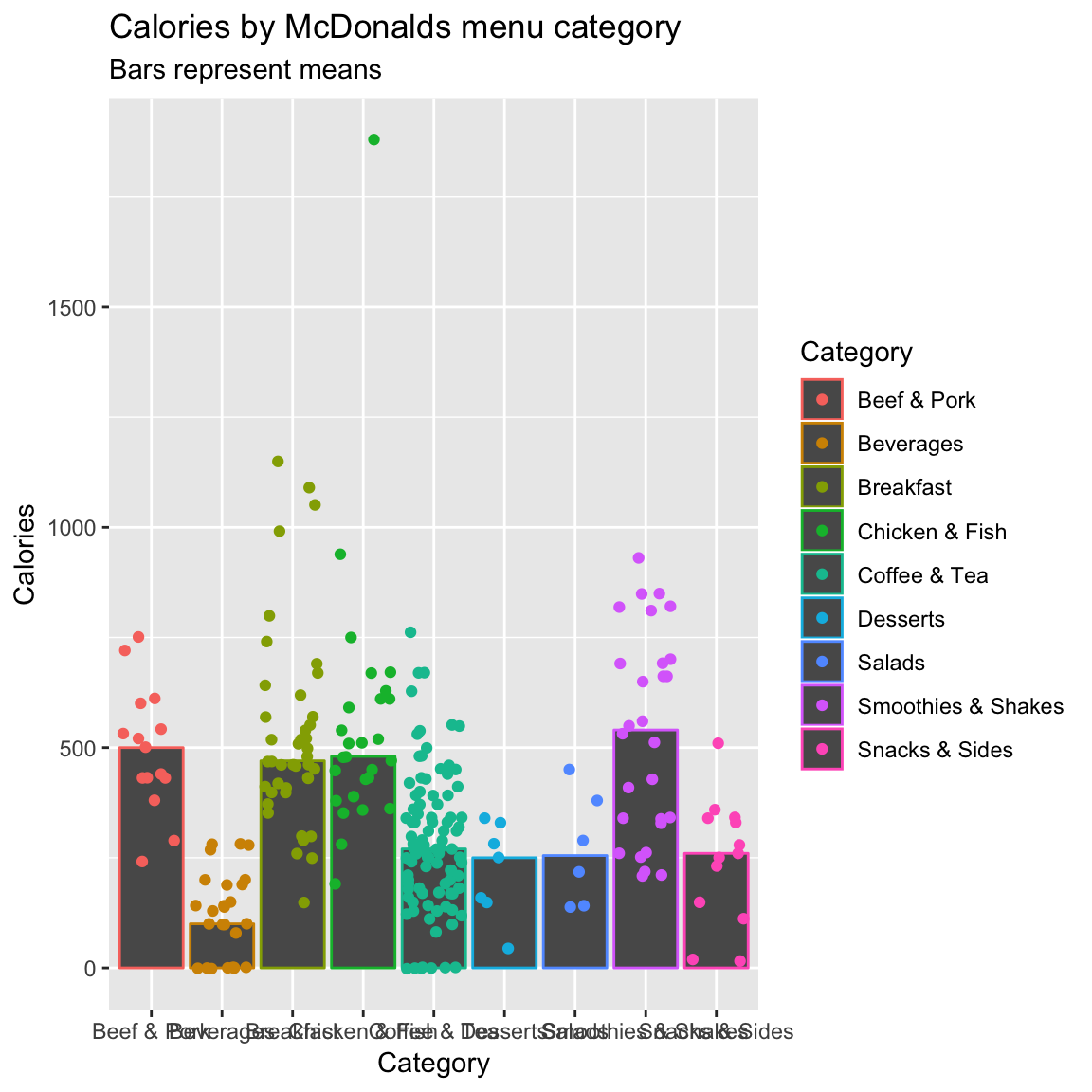1. Save your plot to a .pdf-file called mcdonalds.pdf using ggsave(). When you finish, find your plot in 3_Figures and open it to see how it looks!
# Save mcdonalds_gg to a pdf file
ggsave(filename = "3_Figures/mcdonalds.pdf",
device = "pdf",
plot = mcdonalds_gg,
width = 4,
height = 4,
units = "in")
# Save mcdonalds_gg to a pdf file
ggsave(filename = "3_Figures/mcdonalds.pdf",
device = "pdf",
plot = mcdonalds_gg,
width = 4,
height = 4,
units = "in")
1. Play around with the width and height arguments to change the dimensions of the plot.
# Save mcdonalds_gg to a pdf file
ggsave(filename = "3_Figures/mcdonalds.pdf",
device = "pdf",
plot = mcdonalds_gg,
width = 8,
height = 3,
units = "in")
1. Customize your code to create a jpeg image called mcdonalds.jpeg
# Save mcdonalds_gg to a pdf file
ggsave(filename = "3_Figures/mcdonalds.jpeg",
device = "jpeg",
plot = mcdonalds_gg,
width = 4,
height = 4,
units = "in")

H - Adding labels

Let’s create the following plot with additional point labels using geom_text():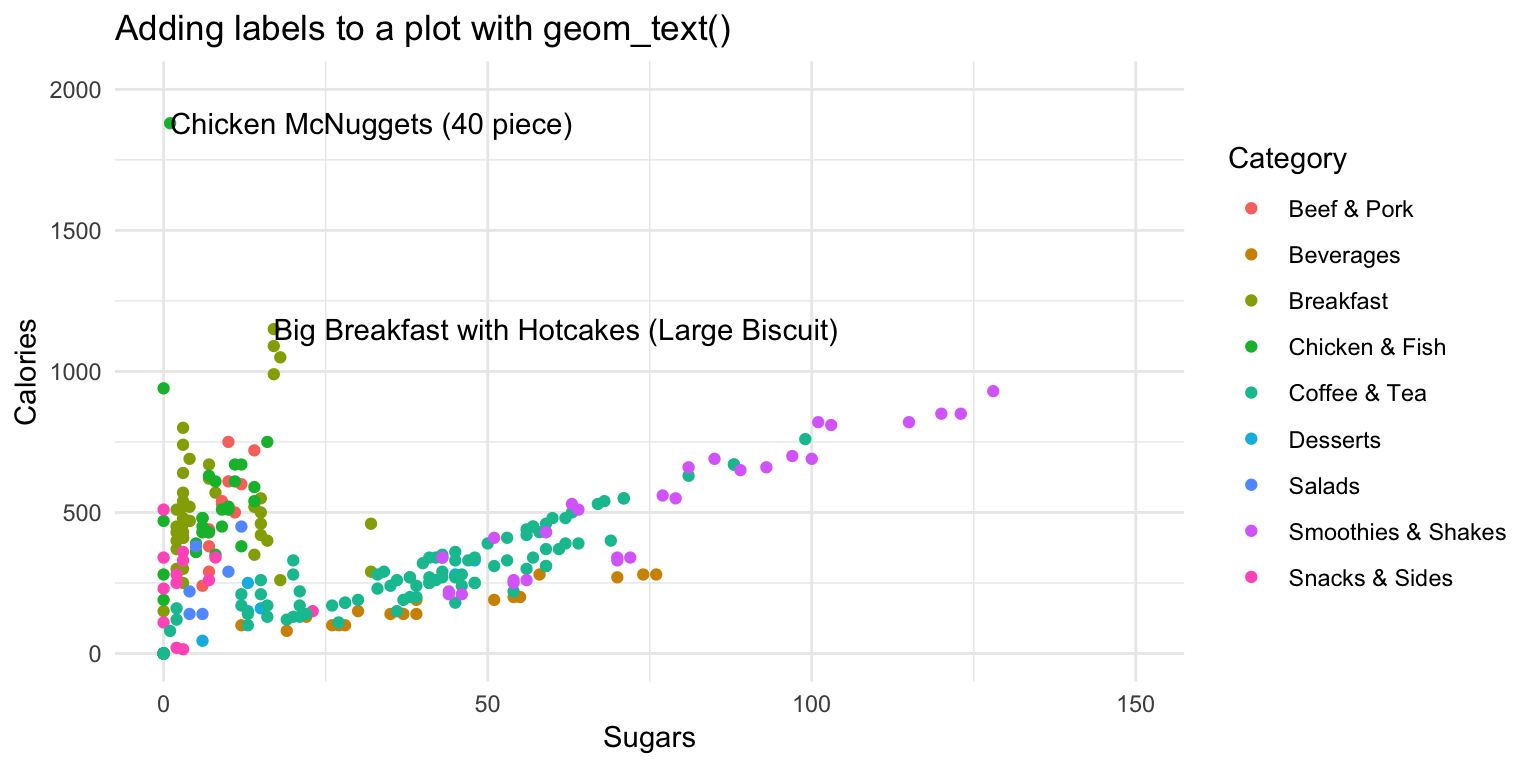ggplot(mcdonalds, aes(x = XX,
y = XX,
col = XX)) +
geom_point() +
xlim(XX, XX) +
ylim(XX, XX) +
theme_minimal() +
labs(title = "XX")
1. Try adding labels to the plot indicating which item each point represents by adding + geom_text().

2. Where are the labels? Ah, we didn’t tell ggplot which column in the data represents the item descriptions. Fix this by specifying the label aesthetic in your first call to the aes() function. That is, include label = Item underneath the line col = XX. Now you should see lots of labels!

3. Customize your geom_text() by including the arguments: geom_text(col = "black", check_overlap = TRUE, hjust = "left").

4. Using the data argument in geom_text(), specify that the labels should only apply to items over 1100 calories (hint: geom_text(data = mcdonalds %>% filter(XX > XX)))

ggplot(mcdonalds, aes(x = Sugars,
y = Calories,
col = Category,
label = Item)) +
geom_point() +
geom_text(data = mcdonalds %>%
filter(Calories > 1100),
col = "black",
check_overlap = TRUE,
hjust = "left") +
xlim(0, 150) +
ylim(0, 2000) +
theme_minimal() +
labs(title = "Adding labels to a plot with geom_text()")1. Play around!
• Specify that the size of the points should correspond to their Calories. Do this with the size aesthetic.

• Instead of mapping Category to the color aesthetic, try creating different facets for each Category with facet_wrap(~ Category).

• Try using a different plotting theme. For example, you can try theme_excel() included in the ggthemes package.

X - Challenges

For these challenges, use the kc_house dataset. Load the data as kc_house

1. Make this plot
• Hint: use scale_color_gradient(low = "green", high = "red"))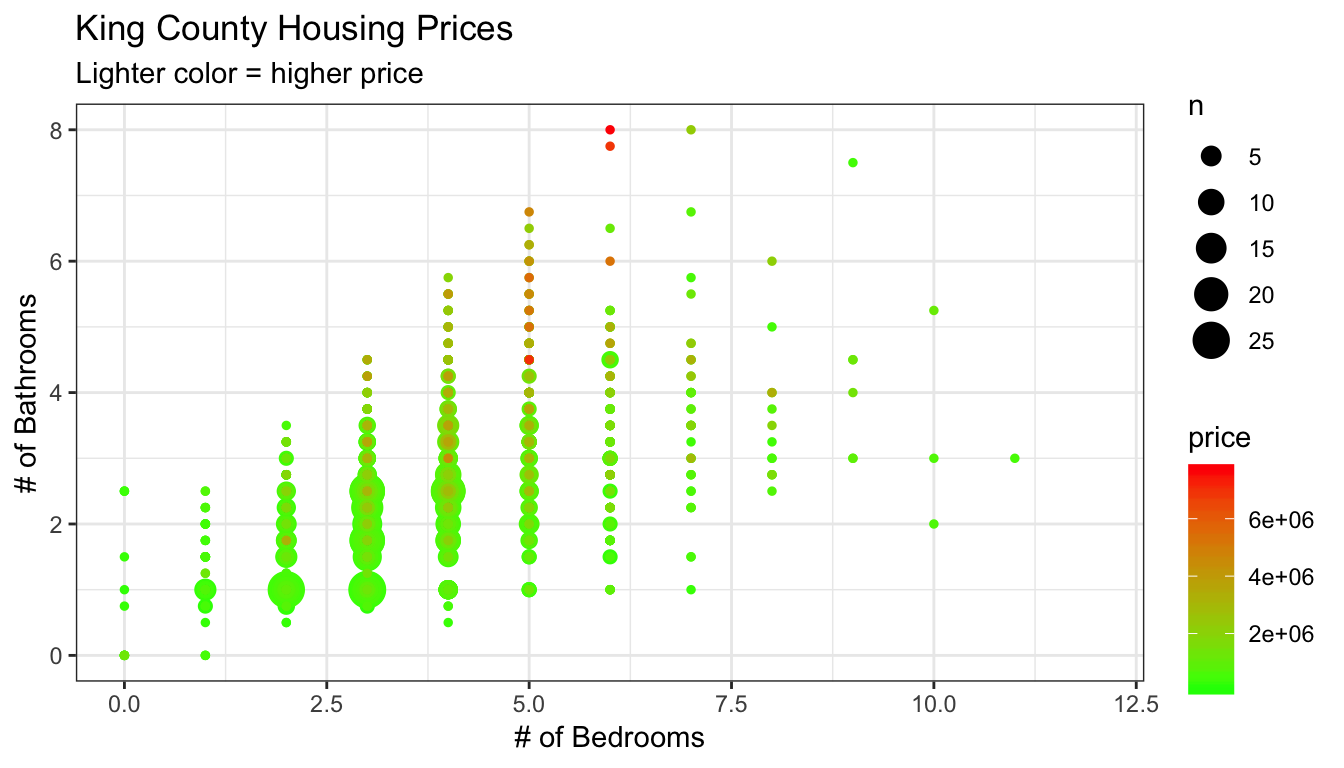ggplot(data = kc_house,
aes(x = bedrooms, y = bathrooms, col = price)) +
geom_count() +
labs(title = "King County Housing Prices",
subtitle = "Lighter color = higher price",
x = "# of Bedrooms",
y = "# of Bathrooms") +
scale_color_gradient(low = "green", high = "red") +
xlim(c(0, 12)) +
theme_bw()1. Make this plot
• Hint: take the log of price with log(price)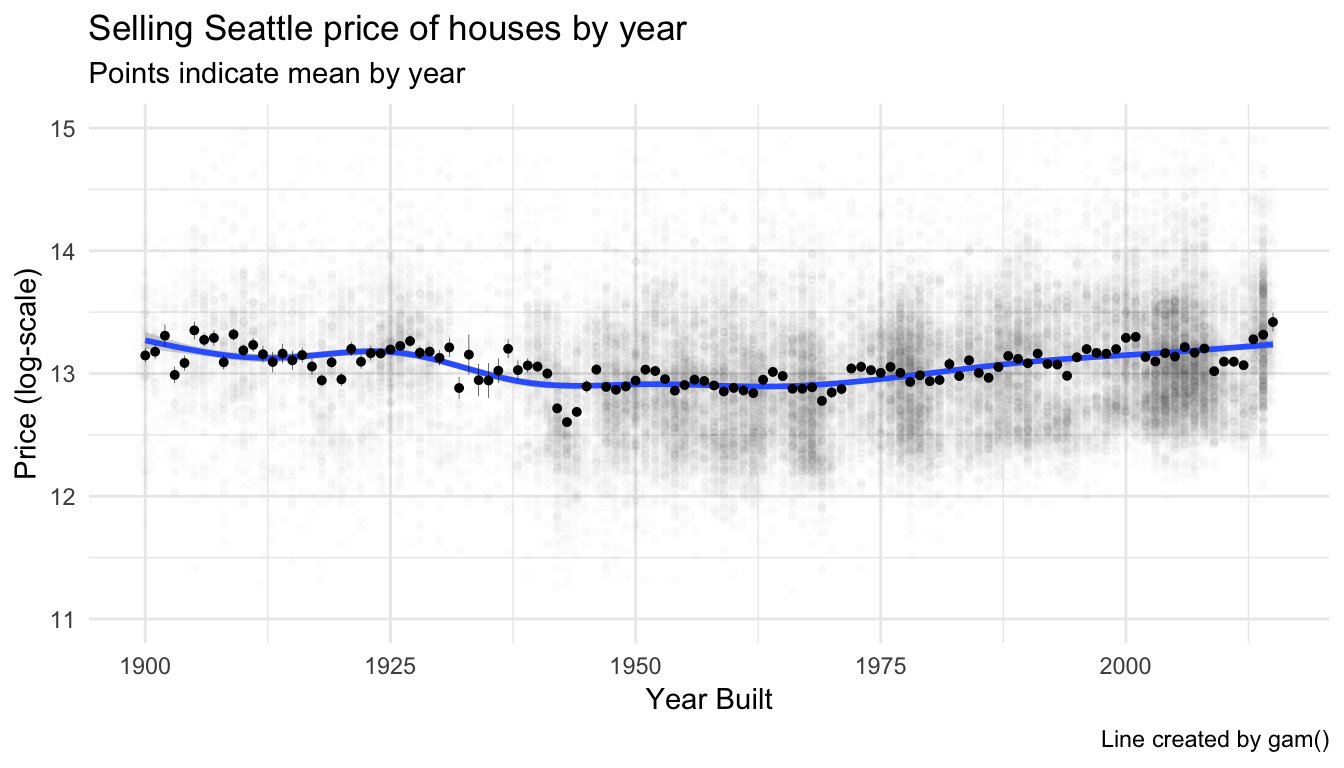ggplot(data = kc_house,
aes(x = yr_built, y = log(price))) +
geom_count(alpha = .01) +
geom_smooth() +
stat_summary(size = .1) +
guides(size = FALSE) +
ylim(c(11, 15)) +
labs(x = "Year Built",
y = "Price (log-scale)",
title = "Selling Seattle price of houses by year",
subtitle = "Points indicate mean by year",
caption = 'Line created by gam()') +
theme_minimal()1. Make this plot
• Hint: Start by creating an aggregated dataset with median home prices of the top 20 zipcodes. Then, use this dataset in ggplot!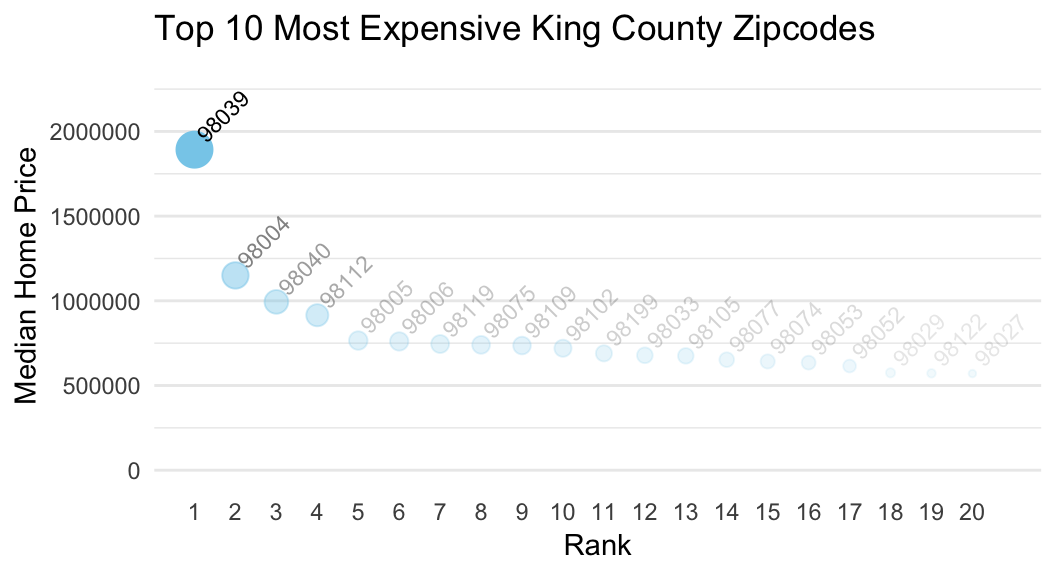agg <- kc_house %>%
group_by(zipcode) %>%
summarise(price_median = median(price)) %>%
arrange(desc(price_median)) %>%
mutate(zipcode = factor(zipcode, levels = zipcode, ordered = TRUE)) %>%
slice(1:20) %>%
mutate(rank = 1:20)

ggplot(agg,
aes(x = rank, y = price_median, label = zipcode, size = price_median, alpha = price_median)) +
geom_point(col = "skyblue") +
geom_text(aes(x = rank, y = price_median), nudge_x = .7, nudge_y = 200000, angle = 45, size = 3, col = "black") +
labs(y = "Median Home Price",
x = "Rank",
title = "Top 10 Most Expensive King County Zipcodes") +
scale_x_continuous(breaks = 1:20) +
ylim(c(0, 2300000)) +
guides(size = FALSE, alpha = FALSE) +
theme_minimal() +
theme(panel.grid.major.x = element_blank(),
panel.grid.minor.x = element_blank())Y - Bonus: Interactive with plotly::ggplotly()

1. With the ggplotly()-function from the plotly package, you can turn any ggplot object into an interactive plot like the one below! Run the following code to see it in action.
# Create a standard ggplot object
MyPlot <- ggplot(data = mcdonalds,
aes(x = Calories, y = TotalFat, col = Category)) +
geom_point()

# Make it interactive with ggplotly()!
library(plotly)
ggplotly(MyPlot)
1. Play around with your plot! See what happens when you hover over the points with your mouse. You can even zoom in by dragging your mouse.

2. Try turning one of your favorite previous plots into an interactive plotly plot using the ggplotly() function!

Examples

# -----------------------------------------------
# Examples of using ggplot2 on the mpg data
# ------------------------------------------------

library(tidyverse)         # Load tidyverse (which contains ggplot2!)

mpg # Look at the mpg data

# Just a blank space without any aesthetic mappings
ggplot(data = mpg)

# Now add a mapping where engine displacement (displ) and highway miles per gallon (hwy) are
# mapped to the x and y aesthetics
ggplot(data = mpg,
mapping = aes(x = displ, y = hwy))   # Map displ to x-axis and hwy to y-axis

#  Add points with geom_point()
ggplot(data = mpg,
mapping = aes(x = displ, y = hwy)) +
geom_point()

#  Add points with geom_count()
ggplot(data = mpg,
mapping = aes(x = displ, y = hwy)) +
geom_count()

# Again, but with some additional arguments
# Also using a new theme temporarily

ggplot(data = mpg,
mapping = aes(x = displ, y = hwy)) +
geom_point(col = "red",                  # Red points
size = 3,                     # Larger size
alpha = .5,                   # Transparent points
position = "jitter") +        # Jitter the points
scale_x_continuous(limits = c(1, 15)) +  # Axis limits
scale_y_continuous(limits = c(0, 50)) +
theme_minimal()

# Assign class to the color aesthetic and add labels with labs()

ggplot(data = mpg,
mapping = aes(x = displ, y = hwy, col = class)) +  # Change color based on class column
geom_point(size = 3, position = 'jitter') +
labs(x = "Engine Displacement in Liters",
y = "Highway miles per gallon",
title = "MPG data",
subtitle = "Cars with higher engine displacement tend to have lower highway mpg",
caption = "Source: mpg data in ggplot2")

# Add a regression line for each class

ggplot(data = mpg,
mapping = aes(x = displ, y = hwy, color = class)) +
geom_point(size = 3, alpha = .9) +
geom_smooth(method = "lm")

# Add a regression line for all classes

ggplot(data = mpg,
mapping = aes(x = displ, y = hwy, color = class)) +
geom_point(size = 3, alpha = .9) +
geom_smooth(col = "blue", method = "lm")

# Facet by class
ggplot(data = mpg,
mapping = aes(x = displ,
y = hwy,
color = factor(cyl))) +
geom_point() +
facet_wrap(~ class)

# Another fancier example

ggplot(data = mpg,
mapping = aes(x = cty, y = hwy)) +
geom_count(aes(color = manufacturer)) +     # Add count geom (see ?geom_count)
geom_smooth() +                   # smoothed line without confidence interval
geom_text(data = filter(mpg, cty > 25),
aes(x = cty,y = hwy,
label = rownames(filter(mpg, cty > 25))),
position = position_nudge(y = -1),
check_overlap = TRUE,
size = 5) +
labs(x = "City miles per gallon",
y = "Highway miles per gallon",
title = "City and Highway miles per gallon",
subtitle = "Numbers indicate cars with highway mpg > 25",
caption = "Source: mpg data in ggplot2",
color = "Manufacturer",
size = "Counts")

Datasets

library(tidyverse)
library(plotly)
library(ggthemes)
File Rows Columns
mcdonalds.csv 260 24

First 5 rows and columns of mcdonalds.csv

Category Item ServingSize Calories CaloriesfromFat
Breakfast Egg McMuffin 4.8 oz (136 g) 300 120
Breakfast Egg White Delight 4.8 oz (135 g) 250 70
Breakfast Sausage McMuffin 3.9 oz (111 g) 370 200
Breakfast Sausage McMuffin with Egg 5.7 oz (161 g) 450 250
Breakfast Sausage McMuffin with Egg Whites 5.7 oz (161 g) 400 210

Functions

Packages

Package Installation
tidyverse install.packages("tidyverse")
ggthemes install.packages("ggthemes")